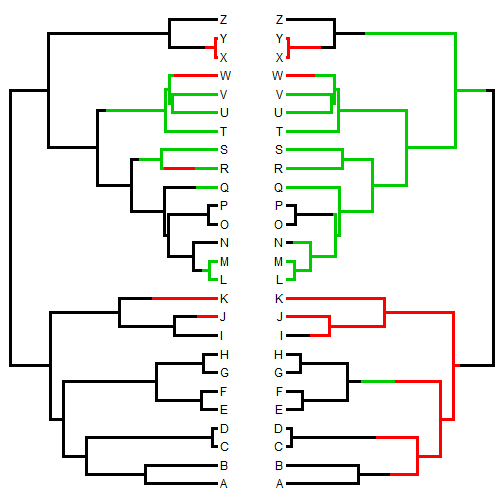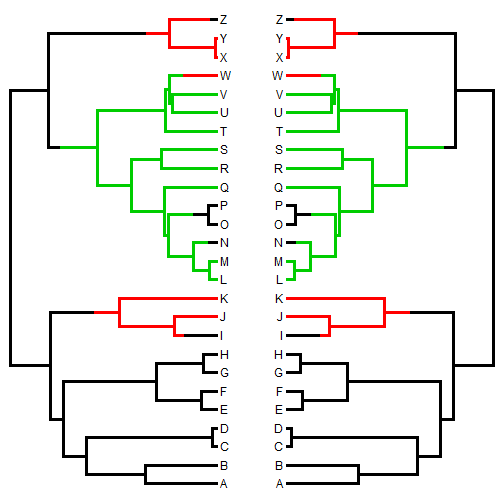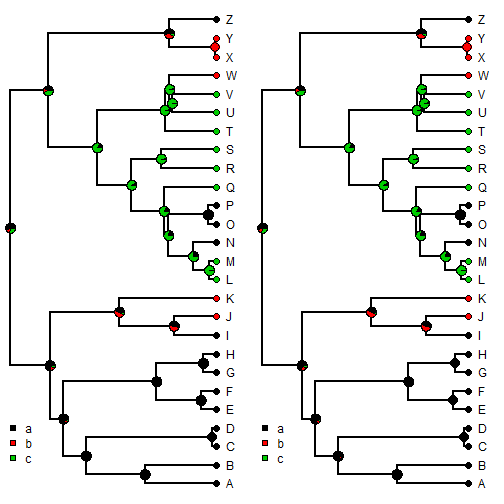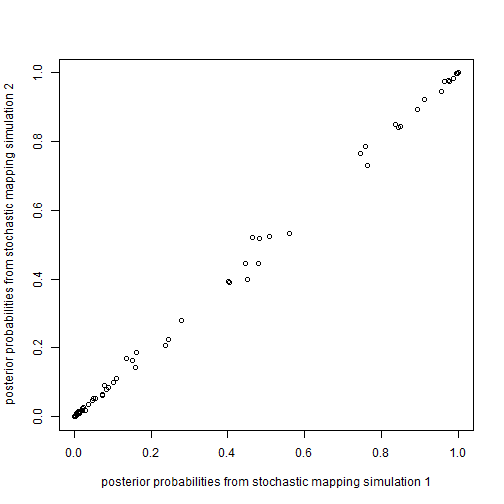## Tuesday, August 4, 2015

### Stochastic mapping: The order of the character data doesn't matter

Today a received the following query about the phytools function `make.simmap` for stochastic charactr mapping:

I had a quick question about the make.simmap function in the phytools package in R. Do the species names from the character trait vector need to be in the same order as the tips from the phylogenetic tree we are using? The order of the species in the character trait vector appears to influence the output when I use the plotSimmap function. Is there a correct way?

The answer is - it certainly shouldn't matter - although it is wise to check as this is, unfortunately, a relatively common bug afflicting PCM functions in R for some reason (e.g., here).

What the user needs to keep in mind, though, is that because stochastic character mapping uses simulations to sample states at nodes and changes in state along edges, the outcome of any particular iteration of the function will differ. This might cause us to (confusingly) suspect that the order of the character vector matters, even though it does not.

Here's what I mean:

``````## use simulated tree & data
library(phytools)
Q<-matrix(c(-2,1,1,1,-2,1,1,1,-2),3,3)
rownames(Q)<-colnames(Q)<-letters[1:3]
tree<-pbtree(n=26,tip.label=LETTERS,scale=1)
tree
``````
``````##
## Phylogenetic tree with 26 tips and 25 internal nodes.
##
## Tip labels:
##  A, B, C, D, E, F, ...
##
## Rooted; includes branch lengths.
``````
``````x<-sim.history(tree,Q)\$states
``````
``````## Done simulation(s).
``````
``````x
``````
``````##   A   B   C   D   E   F   G   H   I   J   K   L   M   N   O   P   Q   R
## "a" "a" "a" "a" "a" "a" "a" "a" "a" "b" "b" "c" "c" "a" "a" "a" "c" "c"
##   S   T   U   V   W   X   Y   Z
## "c" "c" "c" "c" "b" "b" "b" "a"
``````

Now, let's conduct one stochastic mapping simulation with the tip data in the order of the tip labels, followed by a second in which they are in a random order, and see that they are different:

``````x<-x[tree\$tip.label] ## order by tip label
x
``````
``````##   A   B   C   D   E   F   G   H   I   J   K   L   M   N   O   P   Q   R
## "a" "a" "a" "a" "a" "a" "a" "a" "a" "b" "b" "c" "c" "a" "a" "a" "c" "c"
##   S   T   U   V   W   X   Y   Z
## "c" "c" "c" "c" "b" "b" "b" "a"
``````
``````tr1<-make.simmap(tree,x,model="ER")
``````
``````## make.simmap is sampling character histories conditioned on the transition matrix
## Q =
``````
``````##            a          b          c
## a -1.2829525  0.6414763  0.6414763
## b  0.6414763 -1.2829525  0.6414763
## c  0.6414763  0.6414763 -1.2829525
``````
``````## (estimated using likelihood);
## and (mean) root node prior probabilities
## pi =
``````
``````##         a         b         c
## 0.3333333 0.3333333 0.3333333
``````
``````## Done.
``````
``````x<-sample(x) ## randomize order
x
``````
``````##   F   W   Z   A   E   I   N   K   U   J   V   D   L   H   Q   Y   R   C
## "a" "b" "a" "a" "a" "a" "a" "b" "c" "b" "c" "a" "c" "a" "c" "b" "c" "a"
##   T   X   P   O   S   M   B   G
## "c" "b" "a" "a" "c" "c" "a" "a"
``````
``````tr2<-make.simmap(tree,x,model="ER")
``````
``````## make.simmap is sampling character histories conditioned on the transition matrix
## Q =
``````
``````##            a          b          c
## a -1.2829525  0.6414763  0.6414763
## b  0.6414763 -1.2829525  0.6414763
## c  0.6414763  0.6414763 -1.2829525
``````
``````## (estimated using likelihood);
## and (mean) root node prior probabilities
## pi =
``````
``````##         a         b         c
## 0.3333333 0.3333333 0.3333333
``````
``````## Done.
``````
``````par(mfcol=c(1,2))
plotSimmap(tr1,lwd=3)
``````
``````## no colors provided. using the following legend:
##        a        b        c
##  "black"    "red" "green3"
``````
``````plotSimmap(tr2,direction="leftwards",lwd=3)
``````
``````## no colors provided. using the following legend:
##        a        b        c
##  "black"    "red" "green3"
``````These two should be different. For them to be the same we need to control the value of the random number generator seed. Let's try that and see that they are the same:

``````x<-x[tree\$tip.label] ## order by tip label
x
``````
``````##   A   B   C   D   E   F   G   H   I   J   K   L   M   N   O   P   Q   R
## "a" "a" "a" "a" "a" "a" "a" "a" "a" "b" "b" "c" "c" "a" "a" "a" "c" "c"
##   S   T   U   V   W   X   Y   Z
## "c" "c" "c" "c" "b" "b" "b" "a"
``````
``````set.seed(10) ## set the seed
tr1<-make.simmap(tree,x,model="ER")
``````
``````## make.simmap is sampling character histories conditioned on the transition matrix
## Q =
``````
``````##            a          b          c
## a -1.2829525  0.6414763  0.6414763
## b  0.6414763 -1.2829525  0.6414763
## c  0.6414763  0.6414763 -1.2829525
``````
``````## (estimated using likelihood);
## and (mean) root node prior probabilities
## pi =
``````
``````##         a         b         c
## 0.3333333 0.3333333 0.3333333
``````
``````## Done.
``````
``````x<-sample(x) ## randomize order
x
``````
``````##   F   D   H   P   W   R   L   B   A   X   C   S   G   Q   M   J   I   V
## "a" "a" "a" "a" "b" "c" "c" "a" "a" "b" "a" "c" "a" "c" "c" "b" "a" "c"
##   T   U   Z   Y   K   N   E   O
## "c" "c" "a" "b" "b" "a" "a" "a"
``````
``````set.seed(10) ## reset the seed to what it was before
tr2<-make.simmap(tree,x,model="ER")
``````
``````## make.simmap is sampling character histories conditioned on the transition matrix
## Q =
``````
``````##            a          b          c
## a -1.2829525  0.6414763  0.6414763
## b  0.6414763 -1.2829525  0.6414763
## c  0.6414763  0.6414763 -1.2829525
``````
``````## (estimated using likelihood);
## and (mean) root node prior probabilities
## pi =
``````
``````##         a         b         c
## 0.3333333 0.3333333 0.3333333
``````
``````## Done.
``````
``````par(mfcol=c(1,2))
plotSimmap(tr1,lwd=3)
``````
``````## no colors provided. using the following legend:
##        a        b        c
##  "black"    "red" "green3"
``````
``````plotSimmap(tr2,direction="leftwards",lwd=3)
``````
``````## no colors provided. using the following legend:
##        a        b        c
##  "black"    "red" "green3"
``````Finally, there are very few circumstances in which we should be using only one stochastic character maps. That is like just using one sample from a Bayesian MCMC to summarize the posterior distribution. In fact, what we will discover is that if we generate enough samples from the posterior, it doesn't matter what random number seed we start with. The results, integrating over our sample of trees, will be the same.

So, for instance:

``````x<-x[tree\$tip.label]
mtrees1<-make.simmap(tree,x,model="ER",nsim=1000)
``````
``````## make.simmap is sampling character histories conditioned on the transition matrix
## Q =
``````
``````##            a          b          c
## a -1.2829525  0.6414763  0.6414763
## b  0.6414763 -1.2829525  0.6414763
## c  0.6414763  0.6414763 -1.2829525
``````
``````## (estimated using likelihood);
## and (mean) root node prior probabilities
## pi =
``````
``````##         a         b         c
## 0.3333333 0.3333333 0.3333333
``````
``````## Done.
``````
``````## randomize (not that it matters)
mtrees2<-make.simmap(tree,x,model="ER",nsim=1000)
``````
``````## make.simmap is sampling character histories conditioned on the transition matrix
## Q =
``````
``````##            a          b          c
## a -1.2829525  0.6414763  0.6414763
## b  0.6414763 -1.2829525  0.6414763
## c  0.6414763  0.6414763 -1.2829525
``````
``````## (estimated using likelihood);
## and (mean) root node prior probabilities
## pi =
``````
``````##         a         b         c
## 0.3333333 0.3333333 0.3333333
``````
``````## Done.
``````
``````## sumarize the results
mapsum1<-describe.simmap(mtrees1,plot=FALSE)
mapsum2<-describe.simmap(mtrees2,plot=FALSE)
par(mfcol=c(1,2))
plot(mapsum1,cex=c(1,0.7))
prompt=FALSE,x=0,y=4)
plot(mapsum2,cex=c(1,0.7))
prompt=FALSE,x=0,y=4)
````````````plot(mapsum1\$ace,mapsum2\$ace,
xlab="posterior probabilities from stochastic mapping simulation 1",
ylab="posterior probabilities from stochastic mapping simulation 2")
``````OK, I guess this is overkill to simply show that the order of `x` does not matter - but I also do it to emphasize some of the ways stochastic mapping in phytools can be used.

That's all!

1.Hi, Liam.
I was wondering if is possible to incorporate as a input a tip-dated phylogeny in a stochastic mapping analysis?

Thanks

1.Sure, why not. If you can read the tree into R it should work. - Liam

2.Thanks Liam!!

Note: due to the very large amount of spam, all comments are now automatically submitted for moderation.